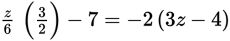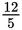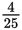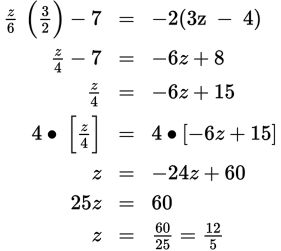# SAT Math Multiple Choice Question 784: Answer and Explanation

### Test Information

Question: 784

4.What value of z satisfies the equation above?

• A. -• B. -• C.• D.Explanation:

D

Difficulty: Easy

Category: Heart of Algebra / Linear Equations

Strategic Advice: Some questions, like this one, are intended to test your fluency with algebraic manipulations, so work carefully and you'll arrive at the correct answer.

Getting to the Answer: Simplify the left side of the equation using multiplication and the right side using the distributive property. Be sure to distribute the negative sign to both terms inside the parentheses on the right side. Next, collect the variable terms on one side of the equation and the constants on the other, and then solve for z using inverse operations: